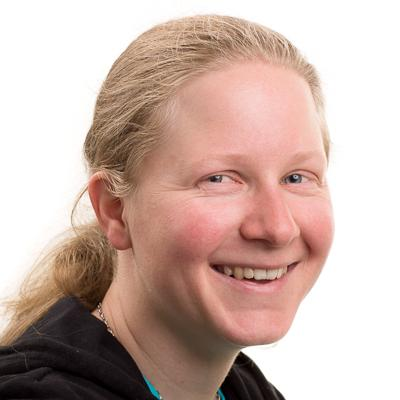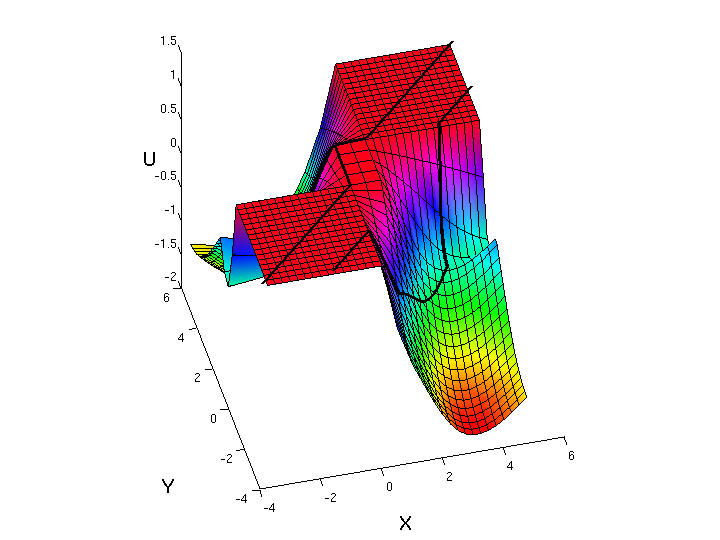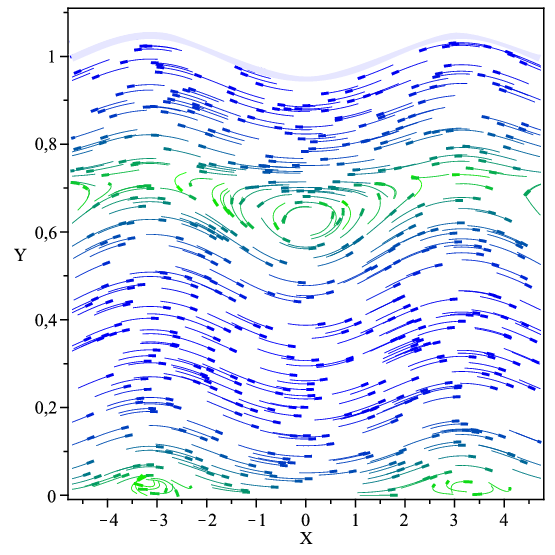# DNA faculty working on PDEsJørgen Endal Jørgen Endal is interested in nonlinear equations of hyperbolic and parabolic type. Such equations include for example a transport term and a diffusion term. In particular, he is working on cases where the diffusion can be nonlocal. The theory needed to treat such equations takes the form of entropy solutions when the equation is hyperbolic, and weak solutions when the equation is parabolic. He has been treating basic questions like existence, uniqueness, and stability of such equations, but also finer properties like regularity and asymptotic behaviour.Mats Ehrnstrom leads a research group investigating equations arising in fluid mechanics, in particular the Euler equations and nonlinear dispersive equations. Of particular interest here are travelling water waves, free-surface flows and rotational currents. A current focus is large-amplitude theory for nonlocal (Whitham-like) dispersive equations. The main interest of the group is on qualitative theory for PDEs, although research in numerical analysis is also pursued.Katrin Grunert is interested in nonlinear partial differential equations that describe wave phenomena, in particular wave breaking, shock formations and stability results.Helge Holden is working on several distinct classes of nonlinear partial differential equations that describe various wave phenomena. In particular, this includes hyperbolic conservation laws, the Camassa–Holm equation, and the nonlinear variational wave equation. In addition, Holden works on equations that model flow in porous media, e.g., flow of hydrocarbons in an oil reservoir, and equations that are completely integrable, e.g., the Korteweg–de Vries equation.Espen R. Jakobsen is working on nonlinear partial differential equations and equations with nonlocal, fractional, or stochastic terms. His research portfolio includes different classes of models such as convection-diffusion, porous medium flow, control theory, stochastic analysis and finance. Typically these problems are degenerate and viscosity or entropy solutions are often involved. Key questions are uniqueness and stability/​approximation of solutions.

## Theory of Partial Differential Equations right barThe solution of the nonlinear variational wave equation utt − c(u)(c(u)ux)x = 0 in transformed variables. From the paper: H. Holden and X. Raynaud. Global Semigroup of Conservative Solutions of the Nonlinear Variational Wave Equation. Arch. Rational Mech. Anal. 201 (2011) 871–964.Travelling waves with a linear vorticity distribution. From the paper: Ehrnström, J. Escher, G. Villari. Steady water waves with multiple critical layers: interior dynamics. J. Math. Fluid Mech. 14(3) (2012) 407–419.